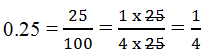## Definition Of Fraction

A fraction is a number that represents part of a whole.

A fraction is written in the form p/q, where q ≠ 0.

A fraction is a way of writing numbers that are not whole numbers.

The top number of a fraction is called the numerator.
The bottom number of a fraction is called the denominator.

### Example of Fraction

1/2 is a fraction, with numerator 1 and denominator 2.
In the figure shown, 7 out of 12 boxes are shaded. The fraction 7/12 names the shaded part.A. 2/3
B. 1/3
C. 0
D. 1/2